# What does refractive error mean?

Figure 3.1 Emmetropia: light from a distant object forms an image on the retina

### Refractive error (ametropia)

‘Refractive error’ (ametropia) means that an eye does not allow light from a distant source to be focused perfectly on the retina. Approximately one-third of the population has a refractive error of more than 1 dioptre, and thus may need spectacles. Myopia is just as common as hypermetropia.

The refractive power of an eye is a function of the corneal curvature (accounting for two-thirds of the power; this cannot be altered) and lens (accounting for one-third of the power; this can be altered by accommodation, provided there is no presbyopia). This is a surprise to most people, since most assume that the lens is the most powerful refractive element. The air–cornea interface is in fact the most powerful refractive element – this becomes quite obvious when you dive into water without any goggles.

Refractive error (ametropia) occurs when the refractive power of the eye does not correlate with the axial length of the eye, so an image from a distant object does not fall on the retina.

### Myopia

‘Myopia’ (short-sightedness) means that the refractive power of the eye is too great relative to the axial length of the eye; as a result, the image of a distant object lies in front of the retina (see Figure 3.2). Therefore, myopia will result if the refractive power is too high or if the eye is too long. Myopia is corrected by a minus (concave) lens, which effectively weakens the refractive power to allow the image to be shifted back on to the retina (see Figure 3.3).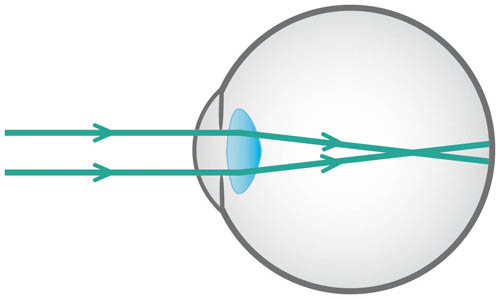Figure 3.2 Myopia: light from a distant object forms an image in front of the retina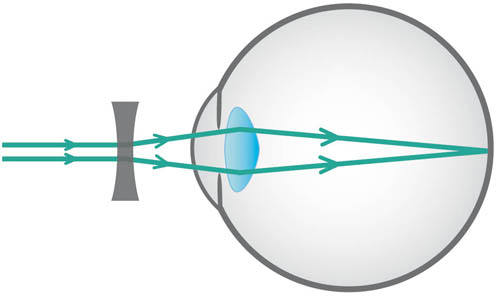Figure 3.3 Myopia corrected by a minus (concave) lens that diverges rays

### Hypermetropia

‘Hypermetropia’ (long-sightedness) means that the refractive power of the eye is too weak relative to the axial length of the eye; as a result, the image of a distant object lies behind the retina (see Figure 3.4). Therefore, hypermetropia will result if the refractive power is too low, or if the eye is too short. Hypermetropia is corrected by a plus (convex) lens, which effectively strengthens the refractive power to allow the image to be shifted forwards on to the retina (see Figure 3.5).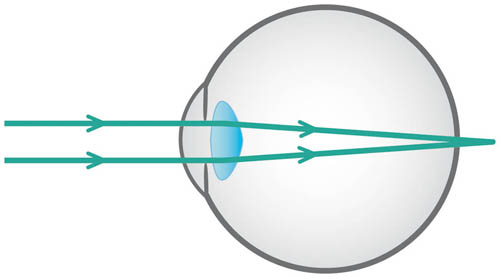Figure 3.4 Hypermetropia – light from a distant object forms an image behind the retinaFigure 3.5 Hypermetropia corrected by a plus (convex) lens that converges rays

### Astigmatism

‘Astigmatism’ refers to the refractive power of the eye being different in different meridians. Therefore, light from a point of a distant object cannot form a single point of an image (see Figure 3.6).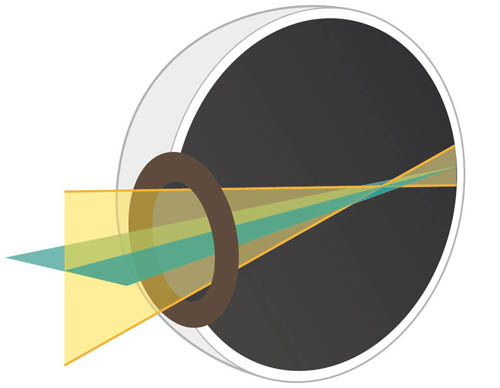Figure 3.6 Astigmatism: light from the same distant point object does not form a single point image, as light is refracted by different amounts in different meridians

An eye with astigmatism behaves as a sphero-cylindrical (toric) lens. The principal meridians form separate line foci and between them is Sturm’s conoid. Where these lines intersect is the circle of least confusion, which is situated at the focal point for the lens spherical equivalent value (see Figure 3.7).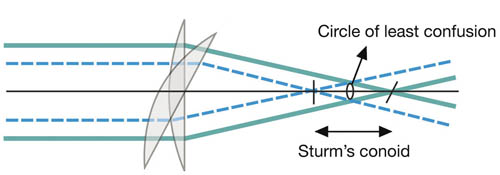Figure 3.7 An eye with astigmatism behaves as a sphero-cylindrical lens, with the circle of least confusion of Sturm’s conoid lying at the focal point for the lens spherical equivalent value

The spherical equivalent of a sphero-cylindrical lens is equal to the sum of the sphere plus half the cylinder (cyl) and is used to establish whether an eye with astigmatism can be considered to be myopic or hypermetropic overall (for more information, see ‘Notation, transposition and spherical equivalent’, p. 17).

This may sound quite complex, but, basically, if an eye with astigmatism behaves as a sphero-cylindrical lens (i.e. a sphere lens with a cylindrical lens superimposed upon it), it follows that to correct astigmatism a sphero-cylindrical lens is required. This is different to myopia or hypermetropia, which can simply be corrected with a spherical lens alone.

The origin of astigmatism is usually corneal, where the corneal curvature and, therefore, the refractive power is different in different meridians. This is why we often explain to patients that astigmatism implies that their eye is shaped like a rugby ball rather than a football.

The degree of corneal astigmatism can be assessed by a keratometer, which gives ‘K’ values for the refractive power in different meridians. The steeper the cornea in a given meridian, the greater the numerical value of the K value for that meridian.

If cataract surgery is proposed, it is important to consider the keratometer K values, since the site of the incision will flatten the cornea in this meridian. By placing the incision on the steepest K meridian the degree of corneal astigmatism is reduced, which can be beneficial to the patient since astigmatism has no refractive advantage.

If astigmatism is present despite having a spherical cornea, it will be due to the lens (lenticular astigmatism). Lenticular astigmatism is eliminated by the placement of a spherical intra-ocular lens implant at the time of cataract surgery.

Some further terms used to describe astigmatism follow.

### Regular astigmatism

This applies when the meridians of maximum and minimum refractive power are perpendicular to each other. This is further divided into:

‘with the rule’ regular astigmatism, in which:

• the cornea is steepest in the vertical meridian and flattest in the horizontal meridian
• the maximal refractive power of the cornea acts vertically (the steepest K meridian will be near 090)
• the weakest refractive power of the cornea acts horizontally (the flattest K meridian will be near 180)
• the axis of a correcting plus lens will be vertical (090), since its power needs to act horizontally to strengthen the relatively weaker horizontal meridian
• this is an example of an eye that has with the rule regular astigmatism: +1.00/+1.50 @ 090. There is a +1.50 dioptre cyl, which has its axis at 090. This cyl is super-imposed on a +1.00 dioptre sphere. In the absence of any lenticular astigmatism one would expect the K value in the vertical meridian to be greater than the horizontal meridian.